## T Network:

Consider two port T Network with assumed voltages V1 and V2 and currents I1 and I2 as shown in Fig. 6.10.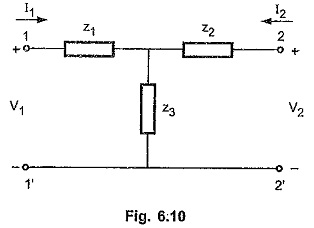Let the z-parameters of the above network are represented as follows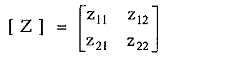We know that, the z-parameters for any network can be calculated by open circuiting input and output terminals ie. by making I1 = 0 and I2 = 0

With the port 2-2′ open circuited :

We have, I2 = 0

Total impedance looking into network from port 1-1′ is equal to ( z1+ z3).

Hence the current I1 in port 1 can be calculated as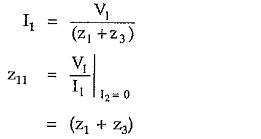The voltage at port 2 ie. V2 can be given as.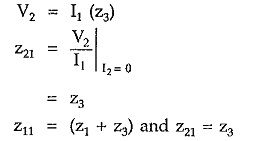With the port 1-1′ open circuited :

We have, I1 = 0

Total impedance looking back into network from port 2-2′ is equal to (z2 + z3) Hence the current I2 in port 2 can be calculated as,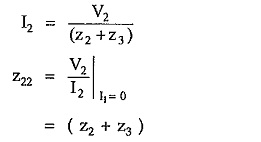The voltage at port 1 ie V1 can be given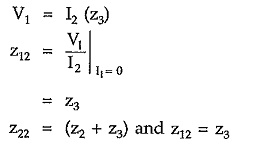Consider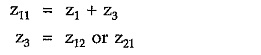Substituting inSubstituting z3 = z12 in above expression,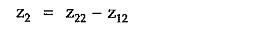Hence, the circuit elements of the equivalent ‘T ‘ network are as follows,

Scroll to Top